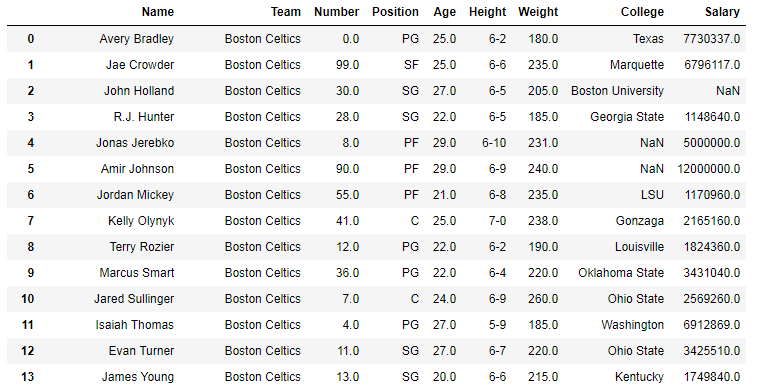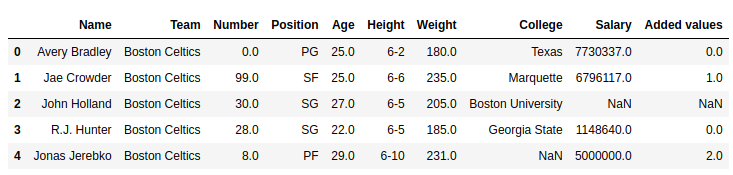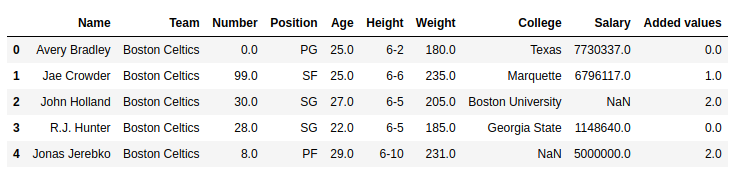# Python | Pandas Series.mod()

Python is a great language for doing data analysis, primarily because of the fantastic ecosystem of data-centric Python packages. Pandas is one of those packages and makes importing and analyzing data much easier.

Python `Series.mod()` is used to return the remainder after division of two numbers

Syntax: Series.mod(other, axis=’columns’, level=None, fill_value=None)

Parameters:
other: other series or list type to be divided and checked for remainder with caller series
fill_value: Value to be replaced by NaN in series/list before operation
level: integer value of level in case of multi index

Return type: Caller series with mod values ( caller series [i] % other series [i] )

In the following examples, the data frame used contains data of some NBA players. The image of data frame before any operations is attached below.Example #1: Checking remainder

In this example, 5 rows of data frame are extracted using `head()` method. A series is created from a Python list using Pandas `Series()` method. The `mod()` method is called on new short data frame and the created list is passed as other parameter.

 `# importing pandas module   ` `import` `pandas as pd  ` `   `  `# reading csv file from url   ` `data ``=` `pd.read_csv(``"https://media.geeksforgeeks.org/wp-content/uploads/nba.csv"``)  ` `   `  `# creating short data of 5 rows  ` `short_data ``=` `data.head()  ` `   `  `# creating list with 5 values  ` `list` `=``[``1``, ``2``, ``3``, ``4``, ``3``]  ` `   `  `# finding remainder ` `# creating new column  ` `short_data[``"Remainder"``]``=` `short_data[``"Salary"``].mod(``list``)  ` `   `  `# display  ` `short_data  `

Output:

As shown in output image, remainder after division of values at same index in caller series and other series was returned. Since nothing was passed to fill_value parameter, the Null values are returned as it is.Example #2: Handling Null Values

Just like in the above example, same steps are done but this time a variable is created and some random value is passed to it. The value is then passed as fill_value parameter to `mod()` method.

 `# importing pandas module   ` `import` `pandas as pd  ` `   `  `# reading csv file from url   ` `data ``=` `pd.read_csv(``"https://media.geeksforgeeks.org/wp-content/uploads/nba.csv"``)  ` `   `  `# creating short data of 5 rows  ` `short_data ``=` `data.head()  ` `   `  `# creating list with 5 values  ` `list` `=``[``1``, ``2``, ``3``, ``4``, ``3``]  ` `   `  `# replacing null value with any number ` `null_replacement ``=` `21218` ` `  `# finding remainder ` `# creating new column  ` `short_data[``"Remainder"``]``=` `short_data[``"Salary"``].mod(``list``, fill_value ``=` `null_replacement)  ` `   `  `# display  ` `short_data  `

Output:
As shown in output image, null values were replaced by 21218 and all operations were done with that value. Hence, instead of NaN, 21218 % 3 = 2 was returned at 3rd position.My Personal Notes arrow_drop_upCheck out this Author's contributed articles.

If you like GeeksforGeeks and would like to contribute, you can also write an article using contribute.geeksforgeeks.org or mail your article to contribute@geeksforgeeks.org. See your article appearing on the GeeksforGeeks main page and help other Geeks.

Please Improve this article if you find anything incorrect by clicking on the "Improve Article" button below.

Article Tags :

Be the First to upvote.

Please write to us at contribute@geeksforgeeks.org to report any issue with the above content.